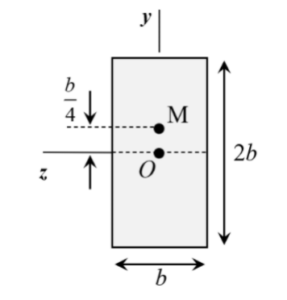# Homework 6.4 (CONCEPTUAL)## 4 thoughts on “Homework 6.4 (CONCEPTUAL)”

1.Chibuzor Vincent Nwaobi says:

How do we find out where the maximum strain is? We are not fully given each dimension.

1.Tejas says:

The problem does not ask you to calculate strains - 6.4 A asks you to compare the magnitude of normal stress at a, b and c. 6.4B asks you to calculate A* and y*.

2.czt says:

Which is the neutral axis/ bending plane? Is it at B for the T shape and and the XZ plane for the block?

3.Kylie Morton says:

Remember that the location of the centroid, and thus the neutral axis, is affected by where the most mass is concentrated. This cross-section has a higher concentration of mass near the top, so the centroid would be shifted upwards.